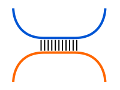Freiburg RNA Tools
Teaching - hybrid-only# Teaching - hybrid-only : max. intermol. bps source at github@BackofenLab/RNA-Playground

The identification of an optimal RNA-RNA interaction without consideration of intramolecular base pairs can be done efficiently in $O(nm)$ time and space for two RNA sequences $S^{1}$ and $S^{2}$ of lengths $n$ and $m$, resp., (Brian Tjaden et al., 2006). For a Nussinov-like interaction prediction, a dynamic programming table $H$ is filled via the prefix-based recursion
$H_{i,j}=\max\begin{cases} H_{i-1,j-1}+1 & \text{if }S^{1}_{i}, \overleftarrow{S^{2}_{j}}\text{ compl. base pair}\\ H_{i-1,j} \\ H_{i,j-1} \end{cases}$,
where an entry $H_{i,j}$ provides the maximal number of intermolecular base pairs for the prefixes $S^{1}_{1..i}$ and $\overleftarrow{S^{2}_{1..j}}$, the reverse sequence of $S^2$. Note, this recursion is a variant of a global Needleman-Wunsch (1970) sequence alignment with special scoring, where only Watson-Crick as well as GU base pairs are considered complementary and thus similiar. A traceback from the entry $H_{i,j}$ with the highest value provides an according optimal interaction.

Here, in order to prepare the computation of accessibility-based interaction prediction, the following interval-based recursion is used to identify the RNA-RNA interaction with the maximal number of intermolecular base pairs. To this end, the four-dimensional table $D$ is filled, where an entry $D^{i,k}_{j,l}$ represents the maximal number of base pairs for the sequence intervals $S^{1}_{i..k}$ and $\overleftarrow{S^{2}_{j..l}}$ under the condition that both interval ends are forming the intermolecular base pairs $(S^{1}_{i},\overleftarrow{S^{2}_{j}})$ and $(S^{1}_{k},\overleftarrow{S^{2}_{l}})$.
A list of up to 15 maximal base pair RNA-RNA interactions is provided via exhaustive traceback for all table entries with maximal value, i.e. $D^{i,k}_{j,l}=\max(D)$.RNA sequence $S^{1}$:
RNA sequence $S^{2}$:
(Computation uses reversed sequence $\overleftarrow{S^2}$)

## Visualization of interacting base pairs (selected structure)

Due to the four-dimensionality of $D$, we only list the optimal hybrid structures (up to 15). On selection, the intermolecular base pairs are visualized.
Possible Structures
Basepair number of selection:
The box provides an ASCII representation of the interacting base pairs of the selected structure with $S^{1}$ on top and $\overleftarrow{S^{2}}$ on the bottom. Note, sequence $S^{2}$ is reversed (running from right ($5'$-) to left ($3'$-end)) within this representation. Note further, if no interacting base pairs are present, no visualization is done.